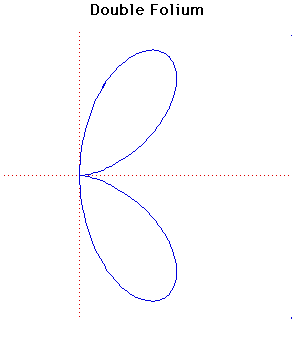# Curves

### Double FoliumCartesian equation:
$(x^{2} + y^{2})^{2} = 4axy^{2}$
Polar equation:
$r = 4a \cos \theta \sin^{2} \theta$

### Description

The general form of the folium is given by the formula
$(x^{2}+y^{2})(y^{2}+x(x+b)) = 4axy^{2}$
or, in polar coordinates
$r = -b \cos\theta + 4a \cos\theta \sin^{2}\theta$.
The word folium means leaf-shaped.

There are three special forms of the folium, the simple folium, the double folium and the trifolium. These correspond to the cases
$b = 4a, b = 0, b = a$
respectively in the formula for the general form.

The graph plotted above is the double folium. There are separate entries for the simple folium and the trifolium.

The double folium is the pedal curve of the tricuspoid where the pedal point is mid-point of one of the three curved sides.

### Associated Curves

Definitions of the Associated curves